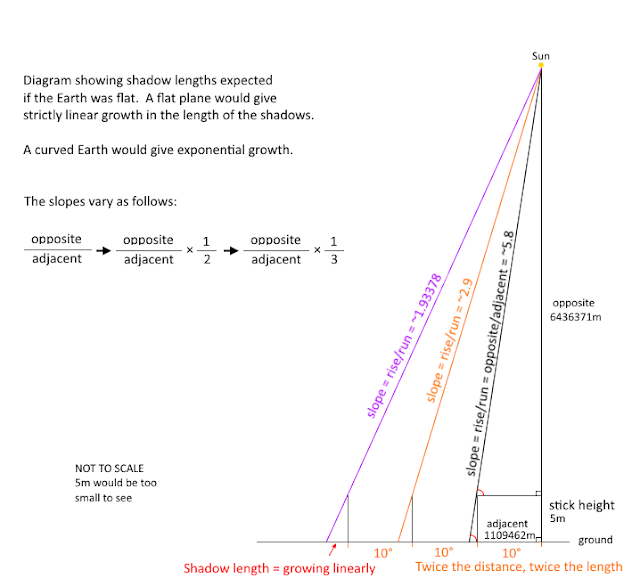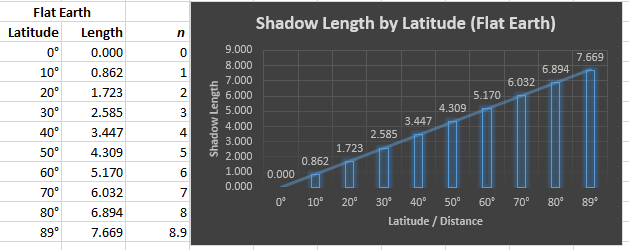This blog is for nonprofit, educational purposes - media is incorporated for educational purposes as outlined in § 107 of the U.S. Copyright Act.

## Sunday, July 24, 2016

### Simple Proof for Convexity of Earth - Flat Earth Disproved

Turns out there is a fairly straight-forward way to prove conclusively that we do not live on a flat plane. Simply pick a line of longitude (along which everyone will experience high noon at the same time, doesn't have to be one perfect line of latitude but each person should measure at high noon on the same day) and measure the length of the sun's shadow at several points of latitude from directly under the sun to as far North as you can (must have at least one measurement that is above 75°).

Requirements For Experiment

• All the sticks should, of course, be very close to exactly the same length (recommend 2 to 5 meters).  I used 5 meters in my example, taller is better.
• The sticks should be placed absolutely plumb (straight up and down).  They cannot lean in any direction.
• The terrain upon which the shadow falls must be flat and level.
• Everyone should be at a similar altitude, this is not as critical as length of the stick - even a few hundred meters wouldn't change the results appreciably.
• Measurement must be taken when the Sun is at the Zenith for that day.  Do not eyeball it, find the exact local time using a calculator such as the USNO calculator and take several measurements to find the shortest one, which will be when the Sun is at the zenith.
• Ideally everyone lies along a single line of longitude and takes the measurements at the same time which eliminates any possibility that the Sun or Earth moving is causing the observed results.
Since you cannot get any of these absolutely perfect this will introduce a small amount of measurement error, so you need to be very precise if you want to perform the experiment and be able to rely on the results.  The further North you can take additional measurements the less likely you will be fooled by measurement error.

ON A SPHEROID

If the Earth is a spheroid then you will find that the measured shadow lengths will follow a power curve that simply cannot be accounted for on a flat plane which will be a linear function.

For example, if we had made these observations on 22, March 2016 at 12:07 UTC along 0° longitude - and a stick length of 5m (let's work in meters) we would find the following shadow lengths for each latitude.

Here are the results:

It is trivial to see here that the shadow length is NOT growing at a linear rate, it is growing exponentially.  You can try it out using the PlanetCalc Shadow Length calculator

And now all you have to do is personally verify that this calculator gives you fairly accurate results for the shadow lengths (I have) and you can be certain that the plane of the Earth simply cannot be flat.

Try this simplified calculator, since it assumes sun is exactly over 0° if it isn't the Equinox you would need to compensate for that.  I'm new to Geogebra so I kept it simple for now.

ON A FLAT PLANE

Now let's compare the expected values above with those on a flat plane, which turns out to be trivial mathematically - we don't even need a calculator.   We just need to calculate the following:

slope = (distance to sun / distance to stick)
length of shadow = (height of stick / slope)

To get this formula we just have to think about two right triangles, using the slope of the first one to figure out the length of the adjacent side of the second one (height/slope).

Our first right triangle is the one from a point directly under the sun at the top of our stick, over to our next stick, say at 10° latitude, which is 1109472m for every 10°s latitude and then up to the sun, finally back down to where we started.  Let's assume the sun is ~4000 miles away or 6437371m.

Since this forms a right triangle we can use "rise over run" to get the slope of our hypotenuse = Opposite/Adjacent, for example for the first 10° we have slope = j/1109462 = ~5.8The second right triangle is the little one formed by our stick and the shadow cast by the above slope.

And the length of our shadow can be found using just height/slope! Since our height is 5m we get: 5/(6437371/1109462) = 0.86 for our first 10° jump.

Let's fill out our table with the values from a flat plane and compare. For each 10° we just multiply our Adjacent side - so we get the formula:  5/(6437371/(1109462×n))What is happening here is that on a flat plane each addition of 10° (or 1109472m distance) gives us a CONSTANT addition to the length of our shadow of 0.861735...

You can see this by comparing any two shadow lengths:

[5/(6437371/(1109462*9))] - [5/(6437371/(1109462*8))] = 0.861735...
[5/(6437371/(1109462*2))] - [5/(6437371/(1109462*1))] = 0.861735...

This linear results simply cannot be fit to the actual data you get for the shadows because the surface of the Earth is curved and indeed, this proves the curvature is positive, so it cannot be concave (or the shadows would get shorter by some power function).

Here is a similar calculator for shadow Length on a Flat Earth, you can verify the distance to the sun and the position of the last stick.

Conclusion

If the data cannot fit your model then that model is falsified - the Flat Earth model here is soundly falsified by a simple measurement that is easily verifiable, even by amateurs, with very inexpensive equipment.

If you want to take some measurements at your latitude and compare them to the PlanetCalc Shadow Length calculator results, make sure you accurately capture all the factors in your video:

• Date
• Exact Time of measurement
• Latitude
• Longitude
• Length of Stick (or Rod)
• Flatness of the Ground
• Plumbness of the Stick at time of measurement

See also 'The Noon Day Project' where children around the world have done this and logged their data.  For example, I took the Fall 2008 Data and converted Latitude values to decimal form and normalized the stick size data (almost all used 100cm) and plotted the resulting shadow lengths.

Not everyone took data on the same day which introduced some error but even in this data you can see the power curve which Excel gives as:  y = 11.608e0.0491x

### An even simpler method?

Apparently this wasn't simple enough for some people so let me try another way to state the exact same thing.

We know from simple geometry that if we take a right isosceles triangle with an object at point (B) at some height (h) and we find the point which is at 45° (C) then we will also be a distance (d) from the point (A) and h will be equal to d at this point.   So when height = distance our angle is 45° ASSUMING STRAIGHT LINES (keep this in mind).  The formula for this is height(h) = distance(d) * tan(angle) and tan(45°) just happens to equal 1.

This also means the line from (B) to (C) has a slope of h/d which is obviously 1.  As shown here.

Now consider point (Z) which is at twice the distance away.  The slope of this line will be 0.5 and we can see this using just trivial division.

We know that SLOPE = RISE(h) / RUN(d)

So that means what we are saying is that DOUBLING THE DISTANCE is EXACTLY the same as taking ONE-HALF THE SLOPE. Mathematically:

This is a BASIC rule of fractions.  And it doesn't matter what the height is or what the distance is.

And to get an angle from slope you simply take the arctan(slope) and arctan(0.5) is 26.565°  You can measure the angle if you prefer.  And don't take my word for it - draw it out and measure it.

NOW...

If the Earth was flat then, with the Sun directly over the equator (90° overhead) at the equinox and at an angle of 45° (slope = 1) above the horizon at latitude 45°N (which I think we all agree is accurate), with TWICE the distance at 90°N we SHOULD find the slope being 1/2 corresponding to a Sun at an angle of about 26.565° -- but this is NOT the case at all! Instead, at 90°N latitude we find the angle to sun to be 0° - lying fully on the horizon!  A HUGE discrepancy with the Flat Model.

This measurement does not and cannot agree with a Flat Earth. This happens on a sphere at 1/4 the distance around. And on a sphere, the latitude of 45°N would be TILTED 45° which means ALL of our true angles point in the SAME direction - towards a distant sun as shown in this diagram.

Now, it's not really EXACTLY 45° - that is ~0.00244° off but this is too small for us to easily measure when you are staring at a blinding sun.

And if you measure the angles at other latitudes you'll find EVERY measurement is consistent with this spherical model and completely incompatible with any Flat Earth model no matter how near or far away you place the sun.

Latitude Observed Angle Of
Sun At Equinox
Distance from
Equator (miles)
Which Height
Is It?
10°
80°
690.3 3915.1
20°
70.01°
1380.0 3793.6
30°
60.01°
2070.3 3587.4
40°
50.01°
2760.7 3291.2
45°
45.02°
3105.2 3107.3
50°
40.02°
3450.3 2897.2
60°
30.03°
4140.0 2393.1
70°
20.04°
4829.6 1761.7
80°
10.09°
5516.5 981.7
89°
1.36°
6119.2 145.3

So we know the Flat Earth claim is FLAT WRONG just from this TRIVIAL geometry.

You can also try to calculate the height of the sun using other distances and angle measurements and you'll find that WILL NOT AGREE, you'll get a different answer for every distance and angle because THE BASE LINE IS NOT STRAIGHT, it is curved.   If the Earth was Flat then EVERY angle and distance would agree and give you the same altitude for the Sun.  It doesn't.

1.You cannot confront dementia with rationality, these data have been available to anyone for centuries, today's flat earthers, like creationists, 9/11 truthers, are impervious to evidence. The only thing to do is to expose the flaws in their theory

1.Yeah I know - been around the block a few times :)

I never expect to change the mind of the person I'm arguing with but rather to give counter information in case anyone reading is honestly looking for answers.

And I find it amusing :)

2.Is your information based on the sun being 93 million miles away from earth?

3.I do not ASSUME the Sun is 93 million miles -- I merely showed here that it CANNOT be over a flat plane.

This is understandable simply by observing the change in angle over distance.

But if Flat Earthers cannot even grasp this TRIVIAL, 5th grade geometry what hope of explaining to them the extremely complex methods required to accurately measure something that is 93 million miles away. Do you have any idea how DIFFICULT that is and how many hundreds of years of effort it took to get to the point where actually could RELIABLY estimate this distance?

The methods and history are WELL documented for anyone who isn't an idiot to research them. People who don't think the Earth is flat don't need that information -- people who do would just ignore it.

2.